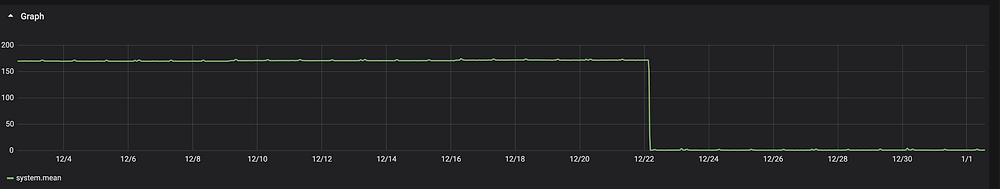# Troubleshooting High Load Average on Linux

Updated: Aug 30

Load average is a key metric to measure CPU performance and system performance on Linux. Today we will learn what system load average means and how to check the high load average on Linux.

What Is system Load in Linux? System load is a measure of the amount of work (meaning the number of currently active and queued processes) being performed by the CPU as a percentage of total capacity. Load averages that represent system activity over time, because they present a much more accurate picture of the state of our system, are a better way to represent this metric.

The load on a system is the total amount of running and blocking process. For example, if two processes were running and five were blocked to run, the system’s load would be seven.

The load average is the amount of load over a given amount of time. Typically, the load average is taken over 1 minute, 5 minutes, and 15 minutes. This enables you to see how the load changes over time.

We can use the following command to get the running process and blocking process. It should be the same as the load average.

`ps -eo s,user,cmd | grep ^[RD] |wc -l`

## Example of Load Average In Linux

A load average of 1.27 on a system with one CPU would mean that, on average, the CPU is working to capacity and another 27% of processes are waiting for their turn with the CPU. By contrast, a load average of 0.27 on a system with one CPU would mean that, on average, the CPU was unused for 73% of the time. On a four-core system, we might see load averages in the range of 2.1, which would be just over 50% of capacity (or unused for around 52% of the time).

So the load average is related to the number of CPUs on our Linux system. For example, load average 20 with 20 CPU is totally different from load average of 20 with 10 CPU.

## Check High System Load average

How to Check Load Average in Linux? We have 4 ways to check the load average on Linux.

• uptime

• w

• top

### Example Of High System Load

Here is one CPU high load example on our production system. The load went over to 170 for one server. The total vCPUs for this server is 64.

After checking, we found that many processes were blocked because of network loss to nfs storage. The system load average number is the same as the number of blocked processes. We fixed the issue after we reboot the server in the end.high system load average on Linuxhigh number of blocked processes

Related Post:

3,892 views